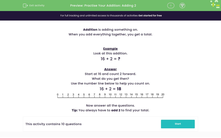Comprehensive & curriculum aligned

In this worksheet, students practise adding 2 to a number to give a total (0-20).Key stage:  KS 1

Curriculum topic:   Number: Addition and Subtraction

Curriculum subtopic:   Add and Subtract to 20

Difficulty level:Worksheet Overview

When you add everything together, you get a total.

Example

16 + 2 = ?

Start at 16 and count 2 forward.

What do you get then?

16 + 2 = 18